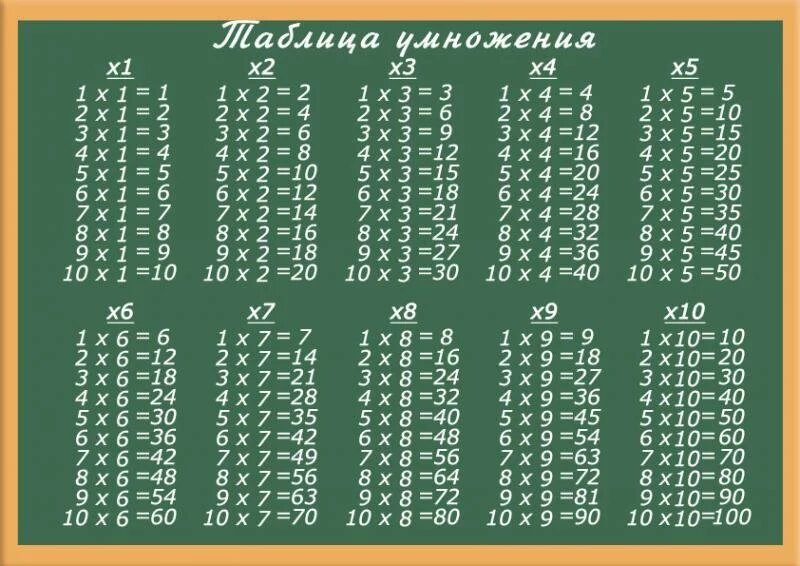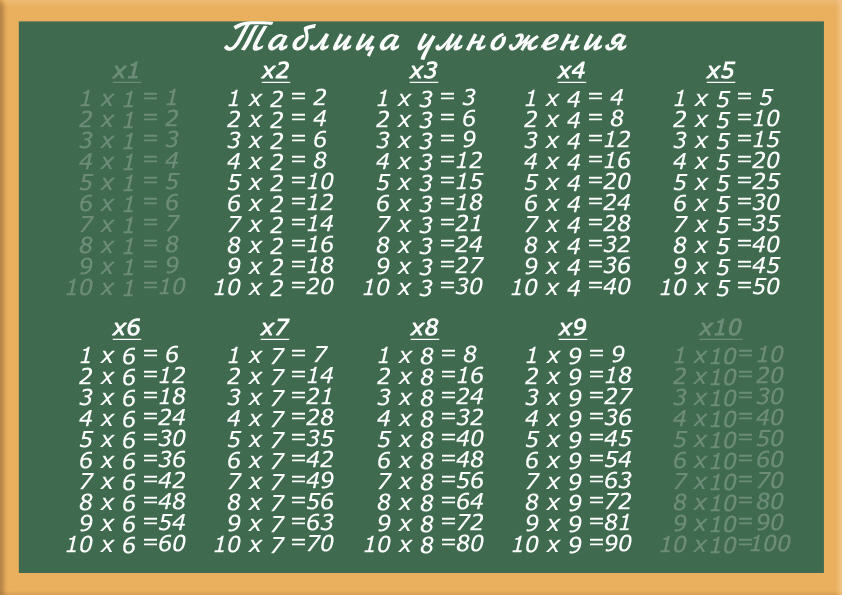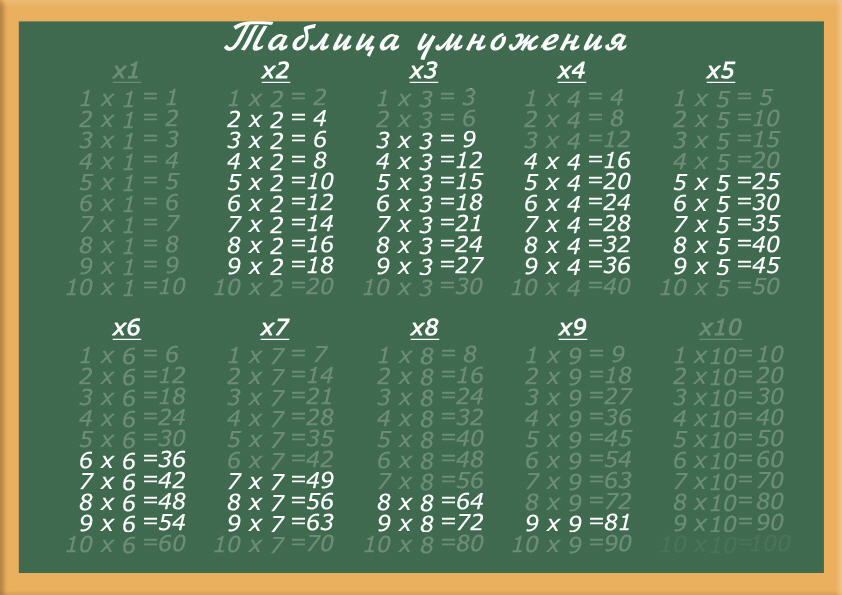# Logical Approach to Learning the Multiplication Table

This is one of the best methods for learning the multiplication table. It not only helps children acquire practical skills but also teaches them to reason and analyze, reducing the number of examples to memorize significantly.The full multiplication table from 1 to 10 contains a total of 100 examples, which can be quite overwhelming to memorize all at once. At the end of this article, we will demonstrate how drastically the number of examples to memorize decreases when applying the logical approach.

## Simplifying the TableMultiplication by 1

This is the simplest rule imaginable. The number remains unchanged when multiplied by one. It's easy to explain and easy to remember.

Multiplication by 10

Another very straightforward rule. When multiplied by 10, simply add a zero to the end of the number. That's it.Commutative Property

Apply the "commutative property" and eliminate duplicates.

The "commutative property" states that changing the order of the factors does not affect the product. Encourage your child to mentally reverse the order of multiplication, i.e., smaller number multiplied by the larger one. This will transform complex examples like 9 * 2 into the simpler example of 2 * 9, which is much easier to remember.

Out of a hundred examples, only 36 remain. to be memorized.

To learn the basic part of the multiplication table from these 36 examples, we suggest using our online simulator in the form of a Pythagoras table

For reinforcement, you can use the following simulator:
Test Your Knowledge of the Multiplication Table